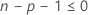# Methods and formulas for model summary statistics in Analyze Binary Response for Response Surface Design

## Deviance R2

The deviance R2 indicates how much variation in the response is explained by the model. The higher the R2, the better the model fits your data. The formula is:

### Notation

TermDescription
DEError Deviance
DTTotal Deviance

The adjusted deviance R2 accounts for the number of predictors in your model and is useful for comparing models with different numbers of predictors. The formula is:

### Notation

TermDescription
R2the deviance R2
pthe regression degrees of freedom
Φ1, for binomial models
DTthe total deviance

While the calculations for adjusted deviance R2 can produce negative values, Minitab displays zero for these cases.

## Akaike Information Criterion (AIC)

Use this statistic to compare different models. The smaller AIC is, the better the model fits the data.

The log-likelihood functions are parameterized in terms of the means. The general form of the functions follow:

The general form of the individual contributions follows:

The following equation gives the form of the individual contributions for a binomial model:

### Notation

TermDescription
pthe regression degrees of freedom
Lcthe log-likelihood of the current model
yithe number of events for the ith row
mithe number of trials for the ith rowthe estimated mean response of the ith row

## AICc (Akaike's Corrected Information Criterion)

AICc is not calculated when.

### Notation

TermDescription
pthe number of coefficients in the model, including the constant
nthe number of rows in the data with no missing data

## BIC (Bayesian Information Criterion)

### Notation

TermDescription
pthe number of coefficients in the model, not counting the constant
nthe number of rows in the data with no missing data
By using this site you agree to the use of cookies for analytics and personalized content.  Read our policy